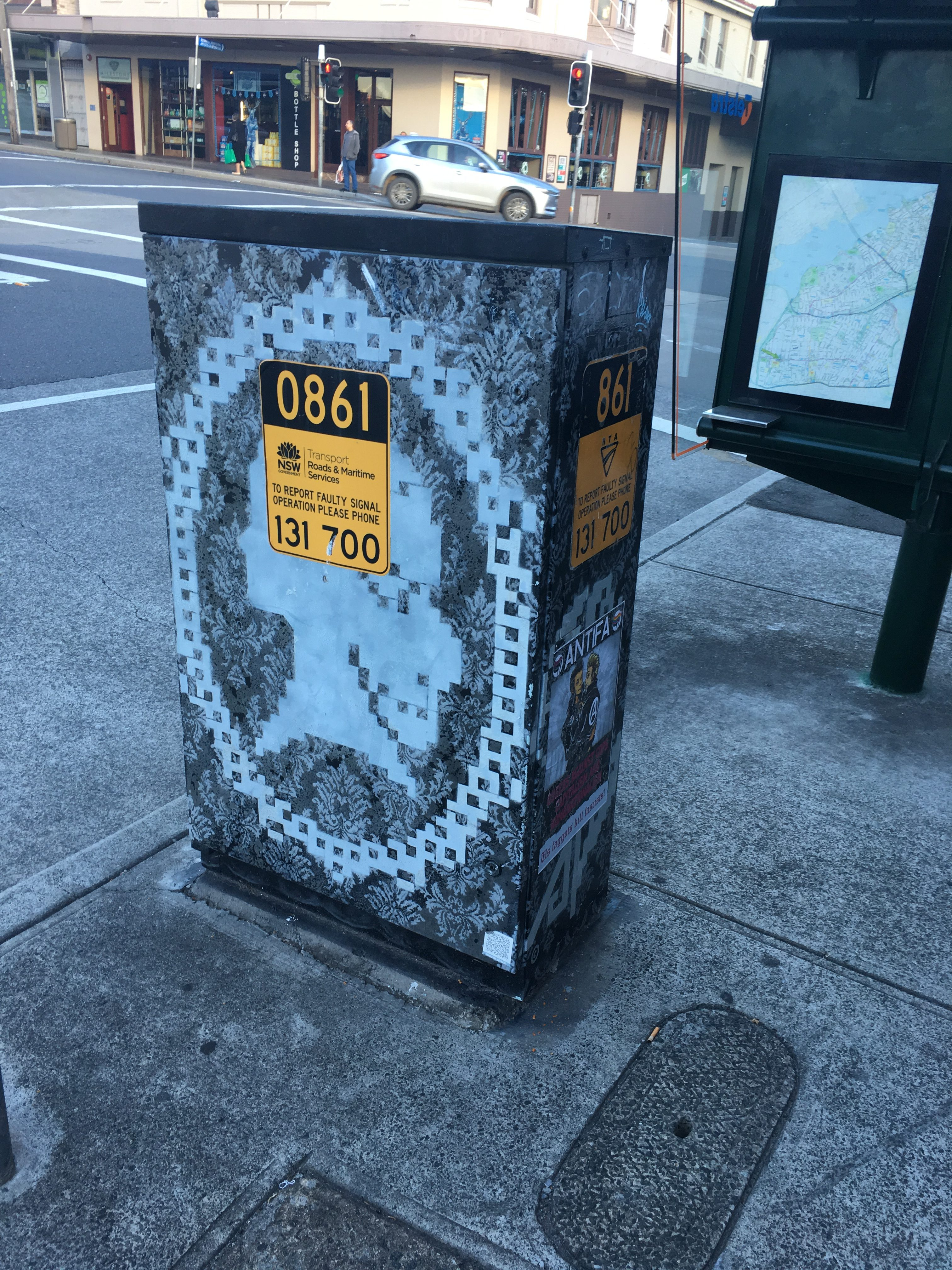# Pedestrians count / Count pedestriansThat which is counted, counts.

The English mathematician and biostatistician Karl Pearson once said,

“That which is measured improves. That which is measured and reported improves exponentially.”

The sorry state of walk signals in Sydney, where a pedestrian should be thankful for 6 seconds of walk time every two minutes at some intersections occurs in large part because we don’t actually systematically count and report the number pedestrians. But TfNSW/RMS has the technology in place to do so, and should. If they counted pedestrians they could in principle consider them in optimisation algorithms that minimised person delay rather than vehicle delay.

There is a simple algorithm which will estimate the number of pedestrians:

• Assume pedestrian groups arrive at an intersection randomly, i.e. each group is independent of each other group. (This is not strictly true, but it is probably true enough, and errors can be calibrated for.)
• Assume intersections are all equipped with beg buttons/actuators.
• Assume actuators only record the first actuation (time stamp) and discard all others. (Hitting the button twice doesn’t matter.)
• Assume someone in the first pedestrian group hits the beg button upon arrival at the intersection, if it is not a “walk” signal. (They may not hit it if there are already people there, but that doesn’t actually matter for this purpose). (Some share of people don’t hit the button on arrival, leading us to underestimate, so again calibration is helpful.)
• Assume we know the average size of a pedestrian group. (On campus, we have estimated about 28% of pedestrians are in groups, near Broadway Shopping Centre, it’s about 33%. Obviously this varies by location and time of day, but can be easily estimated.)

Then, the amount of time between the actuator accepting actuations (beg buttons being begifted begs), and the first beg, is the headway (time) between pedestrians. If there are 2 minute cycles, there are 30 observations per hour, every hour of every day. This should be good enough to get an average.

Example

So for instance, if after the pedestrian actuator accepts actuations, it takes an average of 20 seconds to be actuated, then there are 20 seconds between pedestrian groups per hour (or 180 pedestrian groups). If the average group size is 1.5 people, then there are 270 pedestrians per hour at the intersection.

Data

From what I have been told by those with access about the data TfNSW/RMS collects from their signal systems, they could do this now, and report for every crossing, the number of pedestrians per hour.

I have made a request for this data from the TfNSW OpenData portal to validate and calibrate the model, but that is a black hole and no acknowledgment or response has been provided. Of course, we could all count all the people ourselves, that seems silly, given the data is already being recorded.

Some field calibration and validation of course is required, but this is a relatively small task given the value to be had.